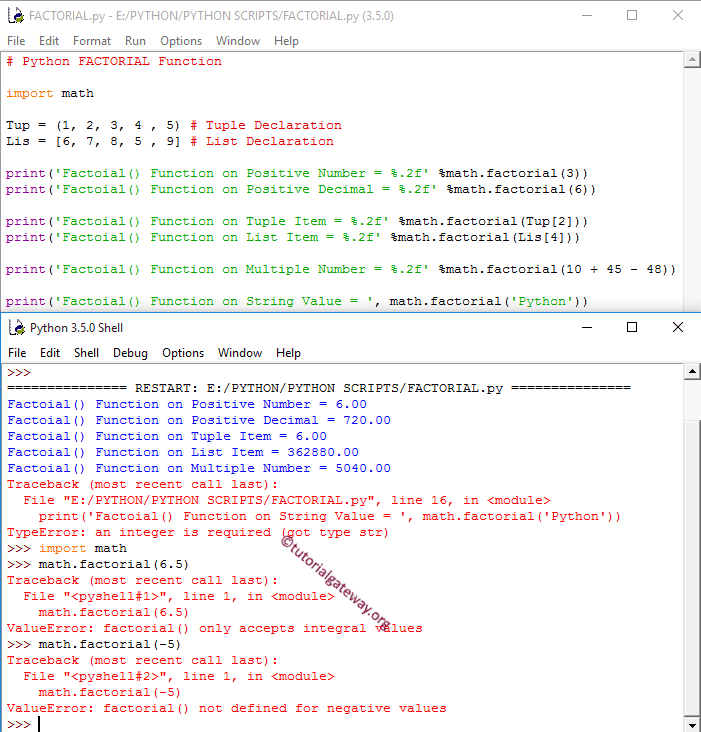# Python factorial

The Python factorial function is used to find the factorial of a specified expression or a specific number. The syntax of the Python factorial function is

`math.factorial(number);`

Number: A valid numerical expression.

• If the number argument is a positive integer, the factorial function returns the factorial of a given number.
• If it is Negative integer, Positive or Negative Decimals, factorial function return ValueError. And if it is not a number, the factorial function returns TypeError.

## Python factorial Function Example

The Python factorial function finds the factorial of a given number. In this math factorial example, we will find the factorial of different data types and display the output.

```# Python FACTORIAL Function

import math

Tup = (1, 2, 3, 4 , 5) # Tuple Declaration
Lis = [6, 7, 8, 5 , 9] # List Declaration

print('Factoial() Function on Positive Number = %.2f' %math.factorial(3))
print('Factoial() Function on Positive Decimal = %.2f' %math.factorial(6))

print('Factoial() Function on Tuple Item = %.2f' %math.factorial(Tup))
print('Factoial() Function on List Item = %.2f' %math.factorial(Lis))

print('Factoial() Function on Multiple Number = %.2f' %math.factorial(10 + 45 - 48))

print('Factoial() Function on String Value = ', math.factorial('Python'))
```1. First, we used the factorial Function directly on Positive integers.
2. Next two statements, We used the factorial Function on Tuple and List items. If you observe the above Python screenshot, this Math function is working perfectly on them.
3. Next, we tried factorial Function directly on multiple values.
4. Here, we used the python factorial on the String value, and it returns TypeError: an integer is required (got type str)
5. Next, We used factorial Function on Decimal value. It returns ValueError: factorial() only accepts integral values
6. Last, We tried factorial Function on Negative value. It is returning ValueError: factorial() not defined for negative values.